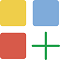# 2.5. Calling Methods that Return Values¶

Formal parameters allow you to pass a value into a method. A method can also pass a value out when it is finished. If a method is a void method, like most of the methods we have seen so far, it does not return a value when called. But some methods return a value when called.

You can see all the methods that are inherited in Turtle in this javadoc (documentation) file. The first column in the method summary indicates the return type of the method. Most of the Turtle methods have a void return type. A void method is often used to modify an object’s attributes. For example, the `forward()` method changes the turtle location, while the `turnLeft()` method changes the direction.

Now look at the methods in the first column having a return type that is not void. The non-void methods tend to start with the word “get” or “is”. Rather than changing an object’s attribute, these methods return an attribute value. For example, the `getXPos()` method will return a turtle’s x position. We refer to non-void methods that return an attribute value as getter methods.

When you use a method that returns a value, you need to save what it returns in a variable or use the value in some way for example by printing it out. The data type of the variable must match the data type of the return value of the method. In the example below the `getWidth()` method returns an int, so we need to assign the result into an int variable `int width = yertle.getWidth();`.

Here are some examples of using getter methods for a particular turtle object. Notice the value returned from each getter method is either stored in a variable or used in a print statement.

```Turtle yertle = new Turtle(world);
int width = yertle.getWidth();
int height = yertle.getHeight();
System.out.println("Yertle's width is: " + width);
System.out.println("Yertle's height is: " + height);
System.out.println("Yertle's x position is: " + yertle.getXPos() );
System.out.println("Yertle's y position is: " + yertle.getYPos() );
```

Note

A common error is forgetting to do something with the value returned from a method. When you call a method that returns a value, you should do something with that value like saving it into a variable or printing it out.Coding Exercise:

Try the code below that changes the turtle’s width and height. How big or small can you make yertle?

(If the code below does not work in your browser, you can also copy in the code below into the Turtle code at this repl.it link (refresh page after forking and if it gets stuck) or download the files here to use in your own IDE.)Coding Exercise:

Fix the errors in the code below so that it prints out the area of the space that the turtle occupies by multiplying its width and height. Remember that you have to do something with the values that the get methods return.

## 2.5.1. toString() Methods¶

Another common method that returns a value is the toString() method. This method is called automatically to try to convert an object to a String when it is needed, for example in a print statement. In the Turtle class, the toString() method returns a String description of the turtle.

```Turtle yertle = new Turtle(world);
yertle.setName("yertle"); // set name before you use toString()
System.out.println(yertle.toString());
// Or you can just use the object here and it will call toString() automatically!
System.out.println(yertle);
```Coding Exercise:

Try some of the get methods and the toString() method in the program below. Note that you have to print out what the get methods return in order to see what they do!

## 2.5.2. Methods with Arguments and Return Values¶

Methods that have argument values and return values are like mathematical functions. Given some input value, the functions computes and returns another value as a result.Figure 1: Method that takes arguments (actual parameters) and returns a value

For example, the Turtle class has a function method `public double getDistance(int x, int y)`. The function computes the distance between the turtle’s current location and the location passed to the x and y formal parameters. You will not write your own methods until Unit 5, but you should be able to trace through method calls that return a value such as the Turtle getter and function methods.

## 2.5.3.Programming Challenge : Turtle Distances¶

1. The Turtle class has a method called getDistance(x,y) which will return the turtle’s distance from a point (x,y). Can you find yertle’s distance from the point (0,0)? Keep in mind the signature for the function `public double getDistance(int x, int y)`. The function returns a double, so you need a variable to store the result.

2. Add another turtle named mertle and make both turtles move. Then find the distance between them. You must use the getXPos() and getYPos() methods as well as the getDistance() method. Ask mertle for their x and y values, then call the getDistance on yertle passing in mertle’s x and y values. Keep in mind getXPos() and getYPos() both return an int.

## 2.5.4. Summary¶

• Some methods return values.

• To use the return value when calling a method, it must be stored in a variable or used as part of an expression. The variable data type must match the return type of the method.